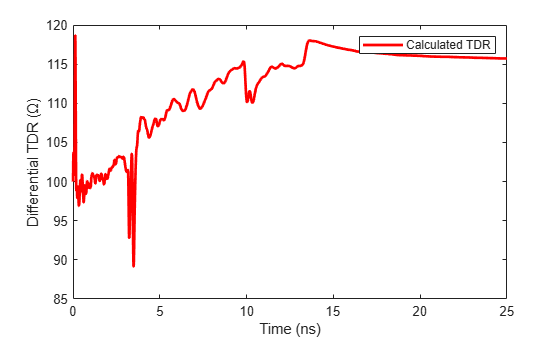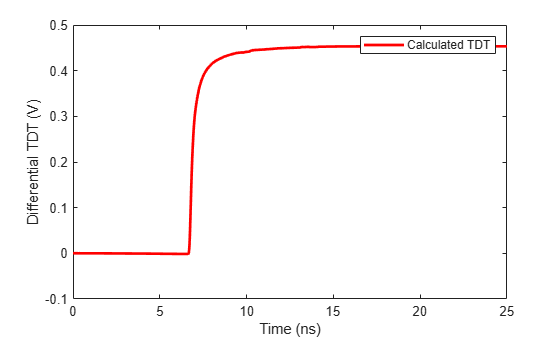# Modeling a High-Speed Backplane (4-Port S-Parameters to Differential TDR and TDT)

This example shows how to use RF Toolbox™ functions to calculate the TDR (Time-Domain Reflectometry) and TDT (Time-Domain Transmission) of a differential high-speed backplane channel.

### Read the Single-Ended 4-Port S-Parameters and Convert Them to Differential 2-Port S-Parameters

Read a Touchstone® data file, `default.s4p`, into an `sparameters` object. The parameters in this data file are the 50-ohm S-parameters of a single-ended 4-port passive circuit, measured at 1496 frequencies ranging from 50 MHz to 15 GHz. Then, get the single-ended 4-port S-parameters from the data object, and use the matrix conversion function `s2sdd` to convert them to differential 2-port S-parameters.

```filename = 'default.s4p'; backplane = sparameters(filename); data = backplane.Parameters; freq = backplane.Frequencies; z0 = backplane.Impedance;```

Convert to 2-port differential S-parameters.

```diffdata = s2sdd(data); diffsparams = sparameters(diffdata,freq,2*z0);```

### Calculate and Plot the Differential Time-Domain Reflectometry

TDR is the reflected voltage signal for a step input. First, extract the differential `S11` data using the `rfparam` function, and convert the `S11` data to TDR voltage transfer function data . Next, create a rational function of that data using the `rationalfit` function, then compute the TDR using the `stepresp` function of the `rfmodel.rational` object. Lastly, plot the calculated TDR.

```s11 = rfparam(diffsparams,1,1); Vin = 1; tdrfreqdata = Vin*(s11+1)/2; tdrfit = rationalfit(freq,tdrfreqdata,'NPoles',350); Ts = 5e-12; N = 5000; % number of samples Trise = 5e-11; % Define a step signal [Vtdr,tdrT] = stepresp(tdrfit,Ts,N,Trise); figure plot(tdrT*1e9,Vtdr,'r','LineWidth',2) ylabel('Differential TDR (V)') xlabel('Time (ns)') legend('Calculated TDR') ylim([0.46 0.55])```### Calculate and Plot the Differential Time-Domain Transmission

TDT is the transmitted voltage signal for a step input. Use the `rationalfit` function to get the rational function object of the TDT voltage frequency data, then use the `stepresp` function to compute TDT. Lastly, plot the calculated TDT.

```delayfactor = 0.98; % Delay factor. Set delay factor to zero if your % data does not have a well-defined delay s21 = rfparam(diffsparams,2,1); tdtfreqdata = Vin*s21/2; tdtfit = rationalfit(freq,tdtfreqdata,'DelayFactor',delayfactor); Ts = 5e-12; N = 5000; % number of samples Trise = 5e-11; [tdt,tdtT] = stepresp(tdtfit,Ts,N,Trise); figure plot(tdtT(1:N)*1e9,tdt(1:N),'r','LineWidth',2) ylabel('Differential TDT (V)') xlabel('Time (ns)') legend('Calculated TDT','Location','best')```### References

 A. S. Ali, R. Mittra. "Time-Domain Reflectometry using Scattering Parameters and a De-Embedding Application" Technical Report, Electromagnetic Communication Laboratory Report No. 86-4, May 1986.